Next: 7.3 6T SRAM Cell Up: 7. Case Studies Previous: 7.1 Power MOS Devices

Subsections

# 7.2 CMOS Inverter

For the investigation of circuit-level degradation a CMOS (complementary MOS) inverter is analyzed. A major advantage of CMOS technology is the ability to easily combine complementary transistors, n-channel and p-channel, on a single substrate. The CMOS inverter consists of the two transistor types which are processed and connected, as seen schematically in Figure 7.10.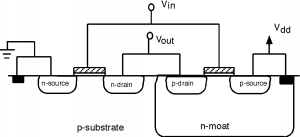The p-channel MOSFET relies on an n-type substrate. As commonly p-type wafers are used for processing, an additional n-type well implant is necessary. In this well, which is a deep region of n-type doping, the p-channel MOSFET is placed. As the p-substrate and the n-well junction is reverse biased, no significant current flows between these regions and the two transistors are isolated.

The output current of the p-channel MOSFET is typically much lower than the current of an n-channel MOSFET with similar dimensions and dopings. This is due to the lower carrier mobility of holes compared to electrons. As the characteristics of the complementary transistors should be as equal as possible, the width of the p-channel MOSFET is typically made larger to compensate the difference. In our example device the necessary geometry factor is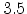to obtain equal drain currents for equal gate biases.Figure 7.11 gives the schematic of the CMOS inverter circuit. It can be seen that the gates are at the same bias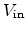which means that they are always in a complementary state. Whenis high,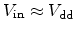, the voltage between gate and substrate of the nMOS transistor is also approximatelyand the transistor is in on-state. The gate-substrate bias at the pMOS on the other side is nearly zero and the transistor is turned off. The output voltage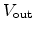is pulled to ground, which is the low state. When the input voltage is in a high-state, the complementary situation occurs and the pMOSFET is turned on while the nMOSFET is turned off. The output voltage is therefore pulled towhich is the high-state. It is important to note that in both states, high and low, no static current flows through the inverter. This is of course only valid when assuming ideal devices with zero off- and leakage-currents.

Considering negative bias temperature instability, the worst stress conditions are imposed on the p-channel MOSFET at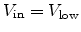. At this bias condition the pMOSFET is turned on, with approximately the same potential at the source and the drain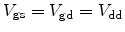and negative gate to substrate voltage.

## 7.2.1 Voltage Transfer Characteristics

The voltage transfer characteristic (VTC) gives the response of the inverter circuit,, to specific input voltages,. It is a figure of merit for the static behavior of the inverter.

The gate-source voltage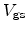of the n-channel MOSFET is equal towhile the gate-source voltage of the p-channel MOSFET calculates as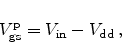(7.1)

and the drain-source voltage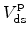of the pMOSFET can be expressed as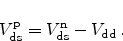(7.2)

Looking at the output characteristics of the two transistors (Figure 7.12), and considering that the drain currents,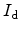, of both transistors must be equal, the voltage transfer characteristic can be extracted, as seen on Figure 7.13. From this figure it is obvious that a shift of the output characteristics of one transistor can have big impact especially around the turn-over point of the VTC.

Figure 7.12: Output characteristics of both transistors up to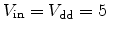V. The resulting drain currents in the inverter circuit must be equal for each.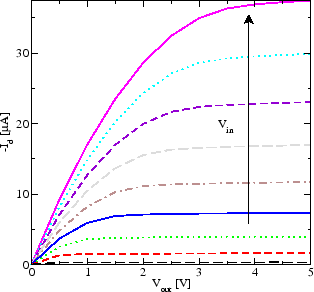n-channel MOSFETp-channel MOSFET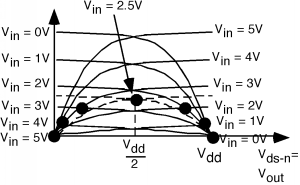The mixed-mode of Minimos-NT allows to simulate the whole circuit while the device characteristics for each device are solved using the semiconductor device equations. Thus, the degradation of the p-channel MOSFET due to negative bias temperature instability can be accounted for in the circuit simulation.The voltage transfer characteristics of the unstressed inverter can be seen in Figure 7.14. The transition from the on to the off state is very well aligned around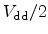.

NBT stress has its highest impact on the p-channel MOSFET during low input. At this condition the transistor has a gate to substrate voltage of approximately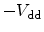. When the circuit is additionally subject to thermal stress, then the threshold voltage of the p-channel transistor is degraded. As the n-channel device has a much lower susceptibility to this type of stress (Section 6.3.7), the circuit loses its symmetry. As shown in Figure 7.15, is the switching point of the output potential moved to a lower input voltage. An interface trap density, which is already a severely damaged interface (Chapter 3), reduces the switching point by more than 1V.## 7.2.3 Transient Behavior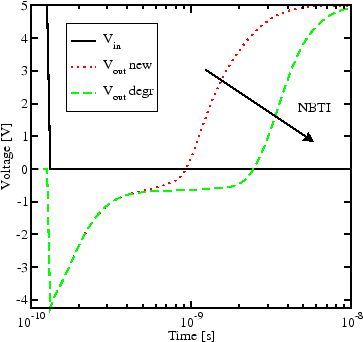Not only in the stationary case does the degradation influence the circuit performance. Transient simulations show (Figure 7.16 and 7.17) that the switching behavior of a circuit comprising a degraded p-channel MOSFET is different. This must be kept in mind when designing timing-critical CMOS circuits.

At high input,, the p-channel MOSFET is turned off and the n-channel device turned on, pulling the output voltage to ground,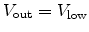. Switching toturns off the n-channel device and on the p-channel device. The switching speed depends on the magnitude of the gate overdrive,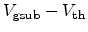. An NBT degraded pMOS transistor has a lower (more negative) threshold voltage, therefore a lower gate overdrive and is turned on slower. The result is a slower CMOS inverter when turning the output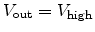, as seen in Figure 7.16.

The opposite case, turning the inverter fromtois completely different, as seen in Figure 7.17. Here, the p-channel transistor is switched from on to off. In this case the gate overdrive equals the magnitude of the threshold voltage. The degraded device, with its more negative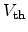is driven into stronger inversion and can, thus, be turned off more quickly.Next: 7.3 6T SRAM Cell Up: 7. Case Studies Previous: 7.1 Power MOS Devices

R. Entner: Modeling and Simulation of Negative Bias Temperature Instability